I worked on the Triangulated Surface Mesh Simplification Package of CGAL in my GSoC 2019 work. Surface mesh simplification -or decimation- is actually pretty self explanatory. Given a surface mesh, try to decrease complexity of the mesh while minimizing the deviation from the original mesh.

## Triangulated Surface Mesh Simplification Framework of CGAL

CGAL already has a framework for surface mesh simplification algorithms. This framework is parametrized by 4 main functional objects obeying following concepts: GetCost, GetPlacement, StopPredicate, and EdgeCollapseSimplificationVisitor. The general algorithm can be summarized as follows:

Lastly, the callbacks defined in the EdgeCollapseSimplificationVisitor concept are called at different stages of the algorithm.

## My Planned and Completed Contributions

Following is the summary table of the contributions that I planned during my application to Google Summer of Code 2019. The table also includes my completion status for each listed item. All of the contributions in this table are explained in detail later in the post.

 # Task Description Status 1 Implementing Garland&Heckbert Simplification This task includes implementing Garland&Hecbert surface mesh simplification as described in this paper using the existing mesh simplification framework of CGAL. completed 2 Comparing our Garland&Hecbert to the Old Method used in CGAL and to Other Libraries This task includes implementing surface mesh simplification using other libraries (namely OpenMesh, libigl, and pmp library); and comparing our results with theirs in terms of runtime and quality. This task also includes comparing our new Garland&Heckbert with the old method used in CGAL, namely Lindstrom&Turk. A new quality comparison metric as described in the Garland&Heckbert paper is also required. completed 3 Documenting the Garland&Heckbert simplification This task includes documenting the newly implemented Garland&Heckbert simplification and providing examples that describe how to use it. completed 4 Parallelizing the Surface Mesh Simplification Framework of CGAL This task includes parallizing the surface mesh simplification framework of CGAL. almost completed

The first big part my CGAL contribution consisted of first three tasks: Implementing Garland&Heckbert, and getting it ready for the production. The second big part of my CGAL contribution was the last task: parallizing the framework.

### 0. Getting to Know CGAL Library

As with any new project, one of the most difficult steps of contributing to CGAL for me was learning the structure of the code. First thing you notice in CGAL is that it is highly templated. I mean, HIGHLY TEMPLATED! The library is so generic that any new eye would be daunted by the extent of it. From the most basic operation of intersecting a plane with a line to the most complex operation of simplifying an entire mesh, you use templates everywhere.

However, once you grasp how the library works, you get amazed by what that genericity provides. I didn’t even touch to the core algorithm while implementing a new version of surface mesh simplification. I only added new files, and plugged things together in a main code. That’s it.

Luckily, CGAL has a lot sources for new developers to ease this initial pain. The first things I did to get on to the development were to read following documents:

1. An Adaptable and Extensible Geometry Kernel: This rather old paper explains the idea of ‘geometric kernel’ used in CGAL. Geometric kernel is the structure that abstracts geometric primitives that users can use in their geometric algorithms. These geometric primitives are objects like points, lines, planes and operations on these objects like checking if two lines intersect or constructing the line that is perpendicular to a plane that goes through a given point. Abstracting objects and operations allows geometric kernel developers to implement different versions of same operations/objects for different needs. For example, one implementation may be more robust, while another implementation may be more efficient, while another implementation may be exact. Algorithms using these operations through kernels are not effected by changes, and the users may choose the kernel they want by changing a single template parameter. The kernel idea presented in the paper is also extensible meaning new operations and objects can be easily added to existing kernels.
2. The Public Developer Manual of CGAL: My next step was hopping into reading the public developer manual of CGAL. Specificially, I read the Introduction, Coding Conventions, Geometry Kernels, Trait Classes, and Checks sections to get into the development fast. I partially checked other sections as needed after delving into the development.
3. Developer Guidelines of CGAL: My next reading before starting on development was the guidelines for developers of CGAL. Initially, I read Building, and Package Directory Structure sections of the guidelines as building the code and understanding the layout of directories can be a little challenging in CGAL in the beginning. Even if it seems like there are unneccessary nested directories, later it becomes clear that it is done to have consistent paths in C++ includes, and CMake files.
4. Surface Simplification Using Quadric Error Metrics: Lastly, I read the paper that I was going to implement in CGAL.

### 1. Implementing Garland&Heckbert Simplification

I implemented Garland&Heckbert simplification using the existing simplification framework of CGAL.

Garland&Heckbert simplification assigns a 4x4 symmetric matrix to each vertex in the mesh. These 4x4 matrices summarizes vertex’s total distance to the faces incident to it. The first 4x4 matrix assigned to a vertex is called the fundamental error quadric. Assume a vertex has spatial embedding $v$ in homogenous form. For each of the planes $% $ incident to a vertex, the distance of $v$ to $p$ is given by $v^Tp$. Given a set of planes around the vertex, the error of vertex induced by these planes is defined by the paper as

The 4x4 matrix $Q$ assigned to $v$ is called the fundamental error quadric. This is the most simple form explained in the paper. However, using only this formula is not good for preserving boundaries of bounded surfaces. Paper suggests penalizing movement of border vertices by adding pseudo-planes for each incident face of the boundary vertex that are perpendicular to incident faces with high penalizing multipliers. My implementation of this final form that handles boundries as well is the fundamental_error_quadric function in GarlandHeckbert_core.h.

The quadric matrix $Q$ assigned to the new vertex created after collapsing an edge that has endpoints $v_1$, and $v_2$ with corresponding quadric matrices $Q_1$, and $Q_2$, is simply the sum of these matrices.($Q = Q_1 + Q_2$) This operation is implemented in the combine_matrices function in GarlandHeckbert_core.h.

Under Garland&Heckbert algorithm, placement of the new vertex after a collapse operation and the cost of the collapse operation are tightly coupled. We solve a single optimization problem to find both the placement and the cost. Given an edge with endpoints $v_1$, and $v_2$ with corresponding quadric matrices $Q_1$, and $Q_2$; the placement $v$, and the cost of the collapse operation is determined by minimizing the function $\Delta(v) = v^TQv$ where $% $ with $a$, $b$, and $c$ being decision variables, and $Q = Q_1+Q_2$. As stated in the paper, since $\Delta$ is quadratic, finding is minimum is a linear problem. Thus, $a$, $b$, and $c$ can be found by solving $\frac{\partial\Delta}{\partial a} = 0$, $\frac{\partial\Delta}{\partial b} = 0$, and $\frac{\partial\Delta}{\partial c} = 0$. This is equivalent to solving the following system where $q_{ij}$ is the element in the $i^{th}$ row, $j^{th}$ column in the $Q$ matrix:

If the matrix is invertible, solution is simply given as

If the matrix is not invertible, paper suggests optimizing the cost on the line segment connecting $v_1$, and $v_2$; and if that fails as well, it suggests choosing either $v_1$, or $v_2$, or the midpoint between $v_1$, and $v_2$. However, it does not provide the explicit math to do so. Therefore, my next step was solving the optimization problem on the line segment connecting $v_1$ to $v_2$.

which is equiavalent to solving

When we plug $v=(1-t)v_1 + tv_2$ into the problem, problem becomes

Even if this seems like a complicated problem, it is a pretty easy one since $Q$, $v_1$, and $v_2$ are constants. Therefore this is just a parabola with 1 variable. Finding the minimum of a parabola in one dimension is a constant time problem. Only thing I had to make sure was checking if the minimum is in the range $[0, 1]$. If not, either $t=0$, or $t=1$ should be minimal.

Formulation above fails at singularities, namely when $(v_2-v_1)^TQ(v_2-v_1) = 0$. In that case, objective reduces to a line segment, and either $v_1$, or $v_2$ is the optimal point. If $v_1^TQ(v_2-v_1)=0$ as well, I simply choose the midpoint.

My final implementation of this optimization is the optimal_point function in GarlandHeckbert_core.h.

With these three core Garland&Heckbert operations -fundamental_error_quadric, combine_matrices, and optimal_point- in hand, I implemented EdgeCollapseSimplificationVisitor, GetCost, and GetPlacement models for the algorithm. GarlandHeckbert_edge_collapse_visitor_base is a model for the EdgeCollapseSimplificationVisitor concept, which populates the state structure that the algorithm uses with fundamental error quadrics. It also updates quadrics of vertices after collapse operations. GarlandHeckbert_cost is a model for the GetCost concept which calculates the cost of collapsing an edge. Lastly, GarlandHeckbert_placement is a model for the GetPlacement concept which computes the optimal placement point for the new vertex created after the collapse operation. I also implemented a trivial stop predicate -GarlandHeckbert_cost_stop_predicate- that runs the algorithm until the minimum cost of collapsing any edge exceeds a given threshold.

### 2. Comparing our Garland&Heckbert to the Old Method used in CGAL and to Other Libraries

Commits: facec019, 587c5f8a, d0dbe88e, 6abd5da9, 592bf5fb, f472c6b1

After completing Garland&Heckbert implementation, my next task was comparing it to the old method used in CGAL, namely Lindstrom&Turk; and to other mesh simplification libraries, namely OpenMesh, libigl, and pmp library. The comparisons had two metrics: runtime, and simplification quality. As simplification quality metric I used two different distance functions. First one was the Hausdorff distance that was already implemented in CGAL. It is a rather fast evaluation metric that tries to measure distance between two meshes with only 1 point. The second distance function is presented in the Garland&Heckbert paper, which tries to measure distance between two meshes by averaging distances of sampled points. Specifically, distance $D$ between meshes $M_i$, and $M_n$, is calculated as follows where $X_i$, and $X_n$ are sampled points respectively from $M_i$, and $M_n$:

where $d(v, M)$ is the minimum distance that point $v$ has to any face of mesh $M$. My implementation of this distance metric can be found in edge_collapse_distance.cpp.

My implementations of OpenMesh simplification can be found in openmesh_polymesh_simplification.cpp, and openmesh_trimesh_simplification.cpp. My implementation of libigl simplification can be found in libigl_simplification.cpp, and my implementation of pmp simplification can be found in pmp_simplification.cpp. My example code for using Garland&Heckbert that I used for comparisons can be found in edge_collapse_surface_mesh_garland_heckbert.cpp, and the example I used for Lindstrom&Turk is the same code with different GetCost, and GetPlacement models.

The results of my comparisons are summarized in the following tables.

Comparison Input Summaries
# Mesh Original Mesh Image Edge Count Edge Count After Simplification
1 man.off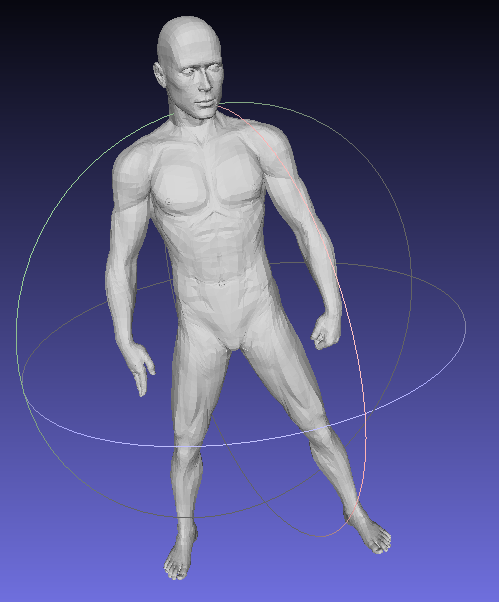52479 5247
2 1255206.stl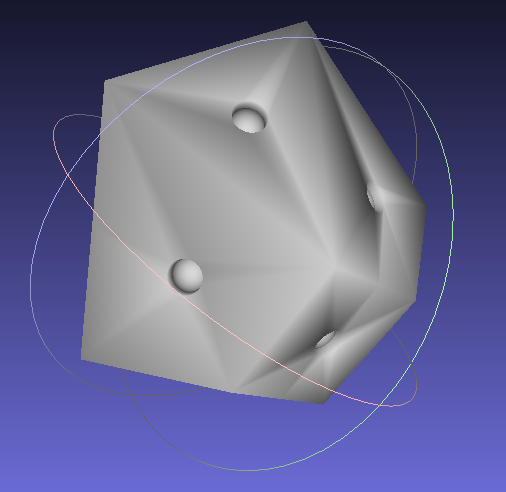1408410 14082
3 472069.stl|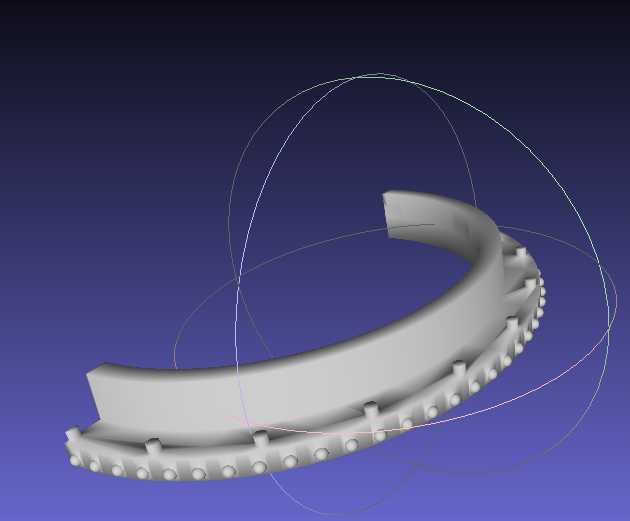183735 9186
Mesh #1(man.off) Simplification Comparison
Simplification Algorithm Resulting Mesh Image Runtime Hausdorff Distance Garland&Heckbert Distance
CGAL Garland&Heckbert (my implementation)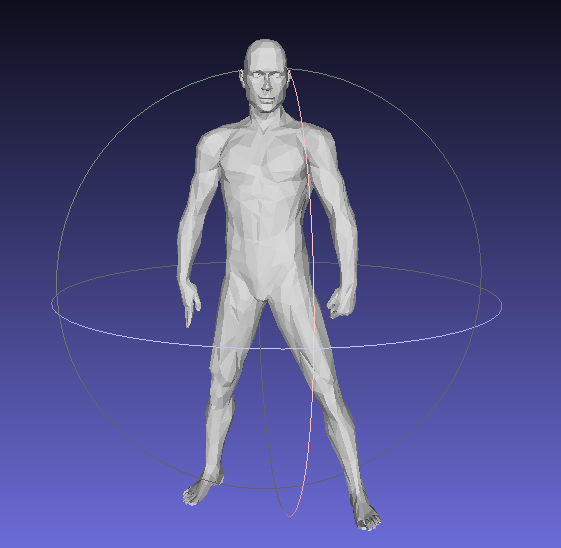439ms 0.00361888 2.70529e-07
CGAL Lindstrom&Turk (old algorithm used in CGAL)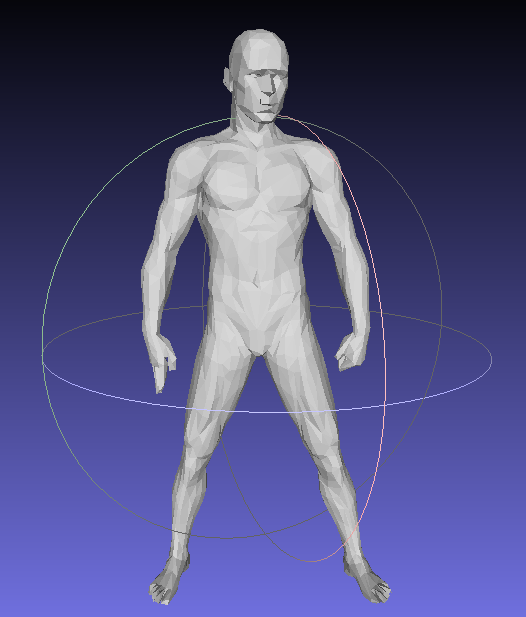1246ms 0.00744421 2.73331e-07
OpenMesh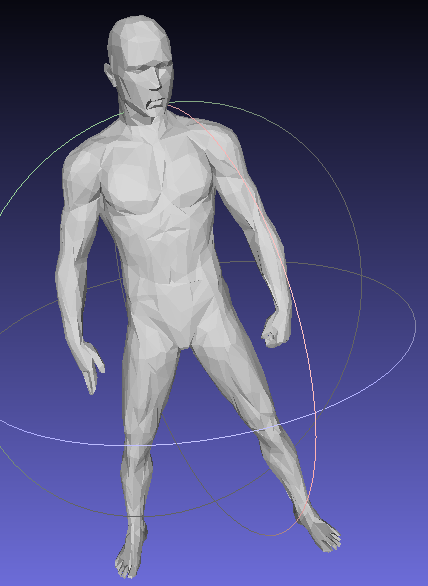156ms 0.00427624 5.86795e-07
pmp library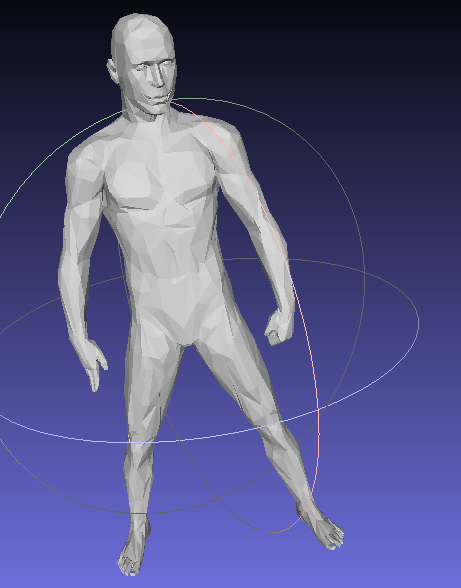355ms 0.00437239 5.23769e-07

In this rather simple mesh (man.off), the fastest algorithm is OpenMesh with 156ms runtime. pmp follows it with 355ms, and our new Garland&Heckbert follows it with 439ms. Linstrom&Turk has a runtime of 1246ms, and libigl gives segmentation fault during read. However, since this mesh is too small in size, it is not a proper input for speed comparison. In terms of quality of the resulting mesh, our Garland&Heckbert results in a mesh with highest quality in terms of both distance functions.

Mesh #2(1255206.stl) Simplification Comparison
Simplification Algorithm Resulting Mesh Image Runtime Hausdorff Distance Garland&Heckbert Distance
CGAL Garland&Heckbert (my implementation)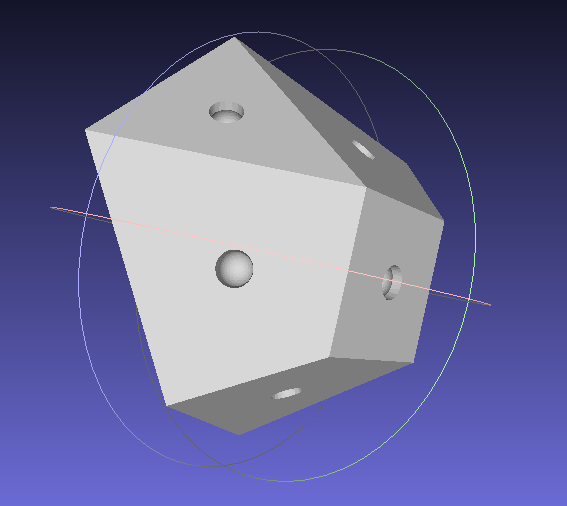18184ms 0.0235652 mesh too big to compute
CGAL Lindstrom&Turk (old algorithm used in CGAL)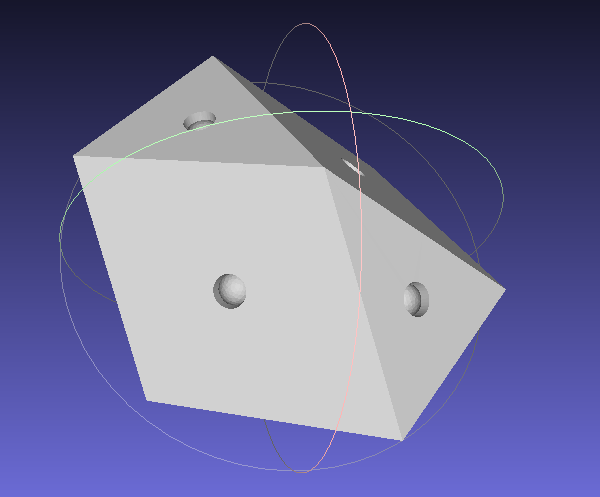71465ms 0.0308491 mesh too big to compute
OpenMesh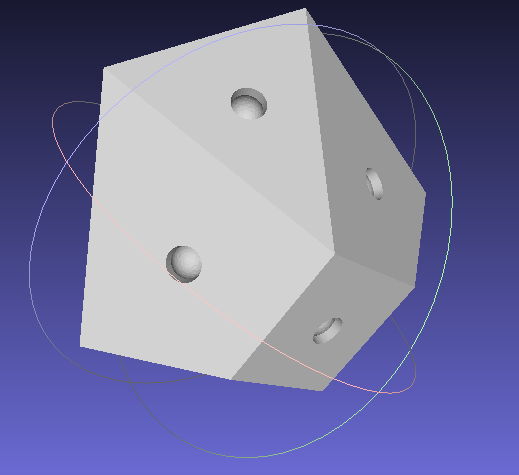11677ms 0.0260296 mesh too big to compute
libigl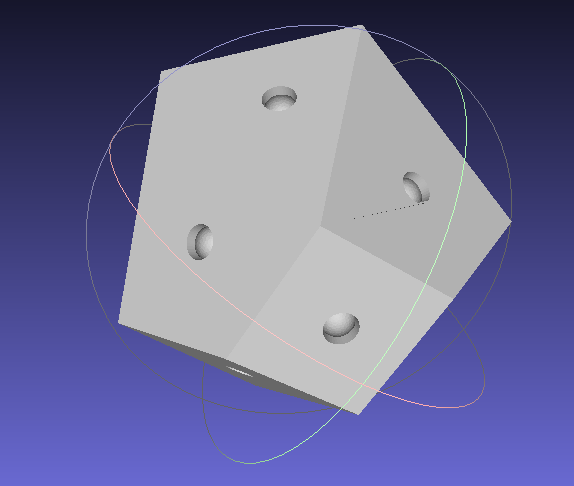31157ms 0.0754579 mesh too big to compute
pmp library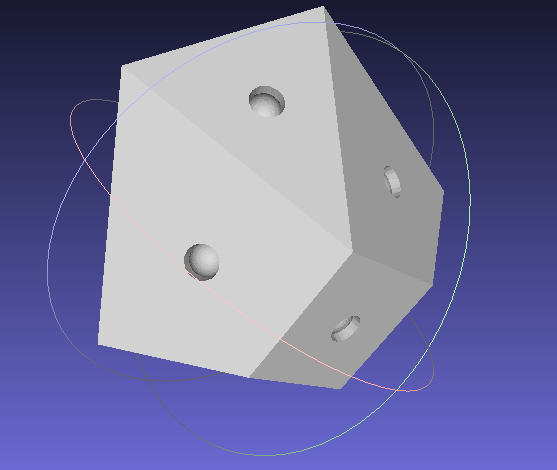34609ms 0.0582223 mesh too big to compute

This example is big enough that speed comparison would be appropriate. Clearly, OpenMesh is the fastest algorithm with 11677ms runtime among the 5 algorithms. Our new Garland&Heckbert comes second with 18184ms. libigl and pmp have runtimes of 31157ms, and 34609ms respectively. CGAL’s old simplification implementation of Lindstrom&Turk comes last with 71465ms. This clearly shows the speed improvement CGAL gets with Garland&Heckbert. Also, our new Garland&Heckbert produces the best result with Hausdorff distance of 0.0235652, while OpenMesh follows it with Hausdorff distance of 0.0260296.

Mesh #3(472069.stl) Simplification Comparison
Simplification Algorithm Resulting Mesh Image Runtime Hausdorff Distance Garland&Heckbert Distance
CGAL Garland&Heckbert (my implementation)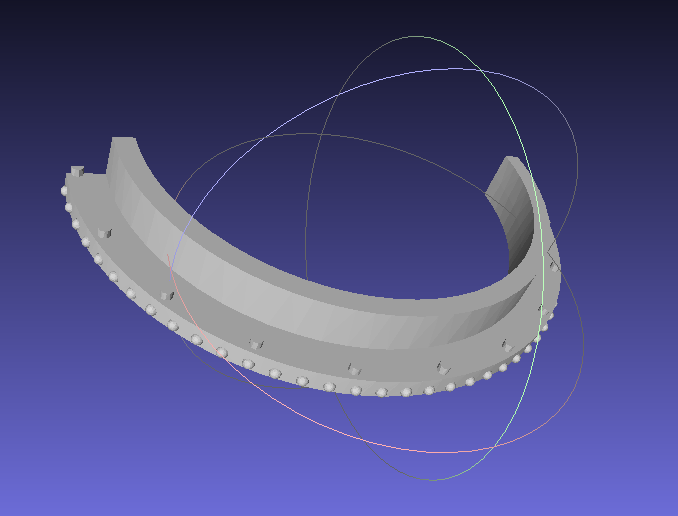1715ms 0.414447 0.000221441
CGAL Lindstrom&Turk (old algorithm used in CGAL)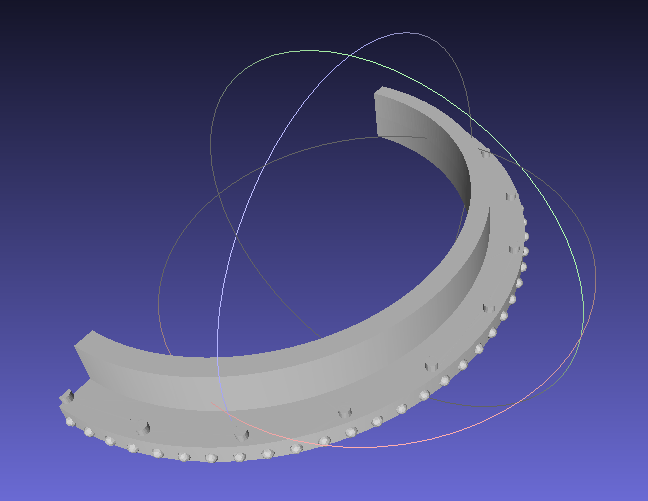4325ms 0.281543 0.000239468
OpenMesh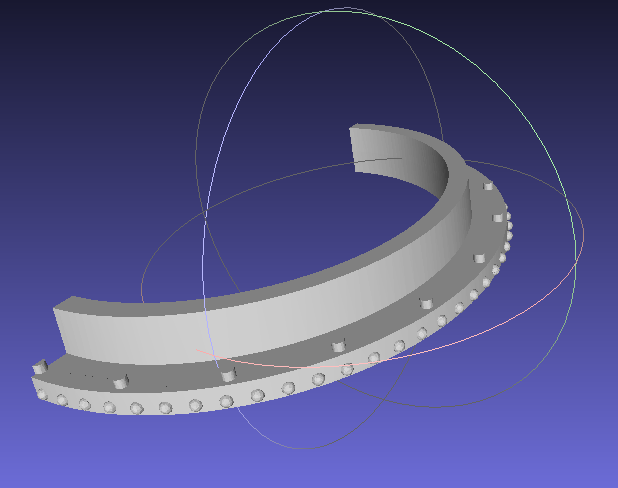898ms 0.399568 0.0010369
libigl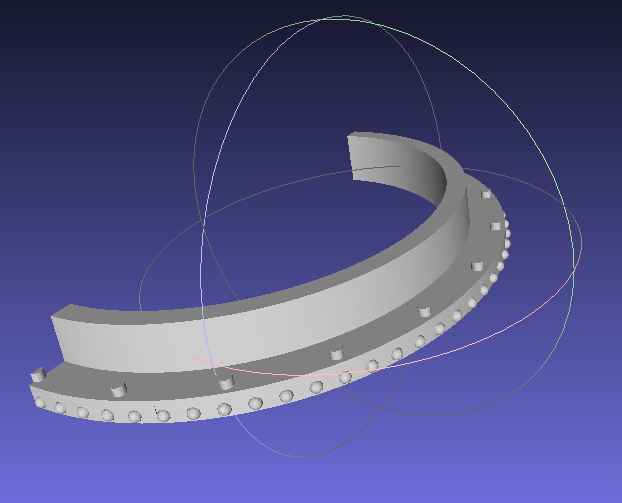3033ms 1.58775 0.000258089
pmp library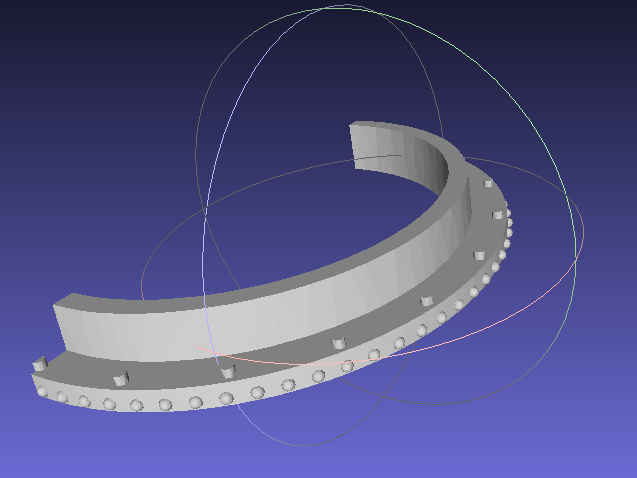1846ms 0.209697 0.00104904

This example confirms our finding that OpenMesh is faster than our new Garland&Heckbert while our new Garland&Heckbert produces more quality results than OpenMesh. Even if both results have similar Hausdorff distances, Garland&Heckbert distance is a better metric (as it averages error over multiple samples instead of using only 1 point), and our Garland&Heckbert has better quality in terms of it.

### 3. Documenting the Garland&Heckbert simplification

Commits: dedb46b6

At this stage, algorithm was complete; and comparisons were done. I have written user and developer documentations for the code I have written. The documentation commit can be found here.

### 4. Parallelizing the Surface Mesh Simplification Framework of CGAL

The next step in my GSoC experience was parallelizing the already existing simplification framework of CGAL. This part was a bit more challenging for me as I was not in the realm defined by the design of simplification package anymore. I was there to change, and update the internals of the algorithm.

I started with learning the parallization approach used in CGAL. CGAL uses Intel TBB as its threading library. I read the complete developer guide of Intel TBB to not have any understanding problems with the library itself. My mentor suggested me to check out the parallization implemented in the Mesh_3 package of CGAL as it resembles the algorithm we are going to implement. Mesh_3 is a mesh generation package that uses Delaunay Refinement to generate 3D meshes.

Parallized mesh generation algorithm in the Mesh_3 package uses a filtered multimap as a priority queue to order refinement operations between different faces or cells. It uses a spatial lock structure to lock some parts of the mesh for synchronization purposes. It also provides some wrapper constructs around Intel TBB, that eases the use of the library, and adds batch job capabilities to it.

My first step for implementing the parallellized version of mesh simplification was to create the structure for distinguishing parallel and sequential versions of the algorithm. Since CGAL is a library, we shouldn’t break any existing code that uses CGAL. The assumption that people has with the simplification package of CGAL is that it is sequential. So, all of the existing code that uses this package should run in sequential mode without any modification. I created the structure that can be found in 3ed639d4 for this purpose.

Next, I adopted the filtered multimap implemented in the Mesh_3 package. it is a structure without update/remove capabilities. So, my first approach was to make it lazy. I supported the structure with an external list, and removed elements lazily during pop operations. My first approach can be found in 1cf02827. However, the result was really slow. Therefore I made it modifiable. The original version had only pushing and popping capabilities. I added update and remove capabilities to it as well. The resulting filtered multimap can be found in Filtered_multimap_container.h.

Surface mesh simplification algorithm in CGAL has two main phases: the collect() phase, and the loop() phase. I parallelized these two steps.

#### Parallelizing the collect() Phase

In this phase, the algorithm creates the priority queue that the loop() is going to use, and populates it with initial costs of collapsing each edge in the mesh. Since this is a read-only operation from the perspective of the mesh, parallelizing it required no real synchronization. I switched sequential collect to parallel collect with a simple task spawning approach for each iteration. My initial implementation can be found in cf289aab. Several updates to this operation exists in other commits but they are generally minor fixes, or fixes due to the priority queue structure update.

#### Parallelizing the loop() Phase

The next step was parallelizing the loop(). The loop() is the main part of the algorithm that collapses edges until the StopPredicate is true. My approach was collapsing a small number of edges in parallel, and waiting for all collapse operations to reach a barrier, and repeating the same thing again. Requiring this barrier with small number of collapses is required since collapsing one edge changes cost of collapsing others. We can’t just collapse everything together. More parallellized we get, more deviation we create with the result of the sequential version. There are two constants that determine the degree of parallelization: number_of_allowed_parallel_collapses, and max_allowed_cost_jump_percentage. As their names suggest, number_of_allowed_parallel_collapses determines maximum allowed number parallel collapses between two barriers and max_allowed_cost_jump_percentage determines the cost ratio between max and min cost edges between two barriers. My implementation of the loop() can be found in 37eea4ac, 0244ab8e.

During collapse operations several threads work on the same mesh structure, and potentially on neighboring edges. Therefore, a synchronization structure is needed. First, I used the same structure that Mesh_3 package uses: Spatial_lock_grid_3. This structure allows threads to lock grid cells in a 3-dimensional grid. I locked 3D embeddings of the two ring of endpoints of an edge. This may seem enough for collapse operation, however during the collapse operation, while one vertex gets removed, the position of other changes. This may create situations where the same vertex gets locked twice by two different threads. To solve this situation, I implemented a new lock structure that works directly on vertices instead of their 3D embeddings. My implementation of this structure can be found in Vertex_lock_structure.h.

## Moving Forward

Even if the parallel version seems complete, I am still dealing with occasional synchronization issues that break the mesh structure. These problems are currently the only things that I couldn’t complete yet. I am currently working on these and I am confident that I will be able to solve them.

CGAL has always been the library that I wanted to use in my projects. However, since it seemed very complicated because of its templated nature, I was always afraid to use it. This summer, I not only used it but became a developer of it. It is probably the most extensive C++ computational geometry library out there and I feel proud to be a part of it. I will continue contributing to CGAL, and use it in my projects.